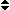Research Group Computational Science and Mathematical Methods (CSMM)

The CSMM (Computational Science & Mathematical Methods) group at SCC does research both in method-oriented mathematics and in mathematical modeling inspired by applications. Mathematical techniques leading to progress in applications, and problems leading to new mathematical concepts are equally important. Furthermore, we promote computational science to the outside (academia, industry and society) by educating university students, high-school students and teachers through our tutorials, projects, theses and our education labs (Simulated Worlds, CAMMP and the Young Persons Lab Mathematics). In general, teaching offered by the CSMM group is as close as possible to - and inspired by - research.

Our method-oriented research focuses on kinetic theory in the broadest sense, i.e. modeling of kinetic equations and related models (e.g. fluid dynamic equations), developing numerical schemes for and using kinetic theory (e.g. Lattice Boltzmann or Uncertainty Quantification). Our application-oriented research aims at bringing modern mathematical techniques into practice. These techniques include modeling, simulation, optimization, inverse problems and uncertainty quantification. In our software implementations we aim to be state-of-the-art and to utilize modern computer architectures.

NameTel.E-MailNameTel.E-Mail